# OliNo

Renewable Energy

## Line Lite International BV Sharp 4W GU10 CW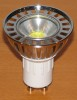Line Lite International BV offers a 230 V led light that looks like a light spot. It is a replacement for existing light spots with a GU10 fitting. Also an Eulumdat file is added in this article.

See this overview for a comparison with other light bulbs.

### Summary measurement data

parameter meas. result remark
Color temperature 5505 K Bright white
Luminous intensity Iv 95 Cd
Beam angle 92 deg
Power P 3.7 W
Power Factor 0.45 For every 1 kWh net power consumed, there has been 2.0 kVAhr for reactive power.
Luminous flux 178 lm
Luminous efficacy 48 lm/W
CRI_Ra 62 Color Rendering Index.
Coordinates chromaticity diagram x=0.3324 and y=0.3428
Fitting GU10
D x H external dimensions 49 x 57 mm External dimensions of the light bulb.
Diameter luminous area 33 mm Dimensions of the luminous area (used in Eulumdat file). This is the diameter of the frosted lens at the front of the light bulb.
General remarks The ambient temperature during the whole set of measurements was 24-27 deg C.

Warm up effect: the illuminance and power decrease with about 20 % during the warm up time and the color temperature increases 8 %.

Voltage dependency: very little dependency of interesting lamp parameters on the voltage.

The Eulumdat file can be found via this link.

Measurement report (PDF)### Eulumdat light diagram

An interesting graph is the light diagram, indicating the intensity in the C0-C180 and the C90-C270 plane. This light diagram below comes from the program Qlumedit, that extracts these diagrams from an Eulumdat file.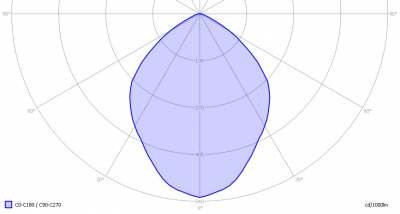The light diagram giving the radiation pattern.

It indicates the luminous intensity around the light bulb. This light diagram is symmetrical around the z-axis.

The unit is Cd/1000lm, meaning the intensity in Cd assuming there would be 1000 lumen in the measured light bulb. This enables comparing different types of light bulbs.

### Illuminance Ev at 1 m distance, or luminous intensity Iv

Herewith the plot of the averaged luminous intensity Iv as a function of the inclination angle with the light bulb.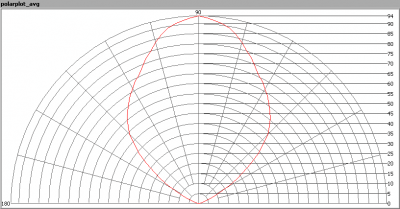The radiation pattern of the light bulb.

This radiation pattern is the same as the one given earlier. This is because in this light bulb’s case, the radiation pattern is symmetric around the z-axis, meaning that the averaged pattern given here is the same as the extraction of the Eulumdat file.

These averaged values are used (later) to compute the lumen output.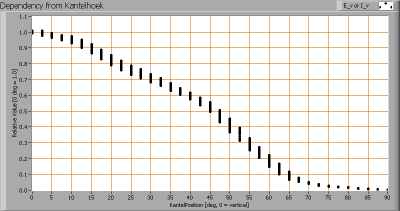Intensity data of every measured turn angle at each inclination angle.

This plot shows per inclination angle the intensity measurement results for each turn angle at that inclination angle. So at 50 degrees inclination angle, all the measurement results of illuminance for all measured turn angles are in the range of 35-45 % of the illuminance value at 0 degrees inclination angle.

When using the average values per inclination angle, the beam angle can be computed, being 92 degrees.

### Luminous flux

With the averaged illuminance data at 1 m distance, taken from the graph showing the averaged radiation pattern, it is possible to compute the luminous flux.

The result of this computation for this light spot is a luminous flux of 178 lm.

### Luminous efficacy

The luminous flux being 178 lm, and the power of the lightbulb being 3.7 W, yields a luminous efficacy of 48 lm/W.

A power factor of 0.45 means that for every 1 kWh net power consumed, a reactive component of 2.0 kVAr was needed.

 Light bulb voltage 230 V Light bulb current 36 mA Power P 3.7 W Apparent power S 8.2 VA Power factor 0.45

### Color temperature and Spectral power distributionThe spectral power distribution of this light bulb.

The measured color temperature is about 5500 K which is neutral to bright white.

This color temperature is measured straight underneath the light bulb. Below a graph showing the color temperature for different inclination angles.Color temperature as a function of inclination angle.

The maximum value of inclination angle is 70 degrees. Beyond that value there is virtually no intensity anymore. The color temperature shows about -8 % deviation from its value at 0 % inclination angle.

### Chromaticity diagram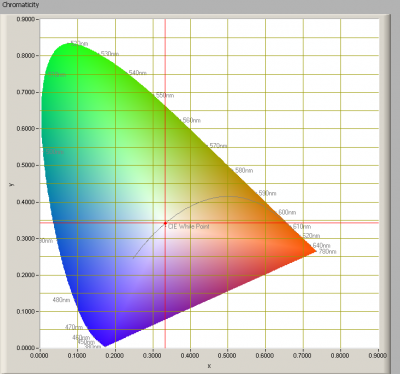The chromaticity space and the position of the lamp’s color coordinates in it.

The light coming from this lamp is on the Planckian Locus (the black path in the graph).

Its coordinates are x=0.3324 and y=0.3428.

### Color Rendering Index (CRI) or also Ra

Herewith the image showing the CRI as well as how well different colors are represented (rendered). The higher the number, the better the resemblance with the color when a black body radiator would have been used (the sun, or an incandescent lamp).

Each color has an index Rx, and the first 8 indexes (R1 .. R8) are averaged to compute the Ra which is equivalent to the CRI.CRI of the light2 of this lightbulb.

The value of 64 is lower than 80 which is considered a minimum value for indoor usage.

Note: the chromaticity difference is 0.0022 indicates the distance to the Planckian Locus. Its value is lower than 0.0054, which means that the calculated CRI result is meaningful.

### Voltage dependency

The dependency of a number of lamp parameters on the lamp voltage is determined. For this, the lamp voltage has been varied and its effect on the following lamp parameters measured: illuminance E_v [lx], color temperature CT or correlated color temperature CCT [K], the lamppower P [W] and the luminous efficacy [lm/W].Lamp voltage dependencies of certain light bulb parameters, where the value at 230 V is taken as 100 %.

The consumed power and the illuminance vary less than 5 % when the voltage varies between 200 – 250 V. When the voltage around 230 V varies with + and – 5 V, then the illuminance varies with less than + and – 0.25 %, which is not visible.

### Warm up effects

After switch on of a cold lamp, the effect of heating up of the lamp is measured on illuminance E_v [lx], color temperature CT or correlated color temperature CCT [K], the lamppower P [W] and the luminous efficacy [lm/W].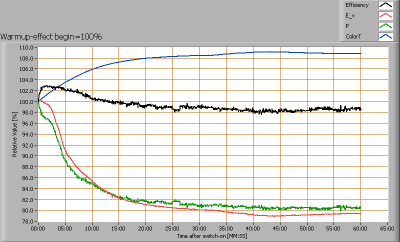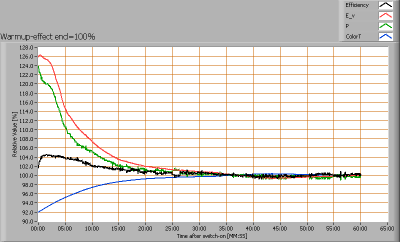Effect of warming up on different light bulb parameters. At top the 100 % level is put at begin, and at bottom at the end.

The illuminance and the power decrease 20 % during the warmup time of 25 minutes and the color temperature increases with 8 % during that time.